# Rank the following organic compounds from most soluble in water to least scluble in water.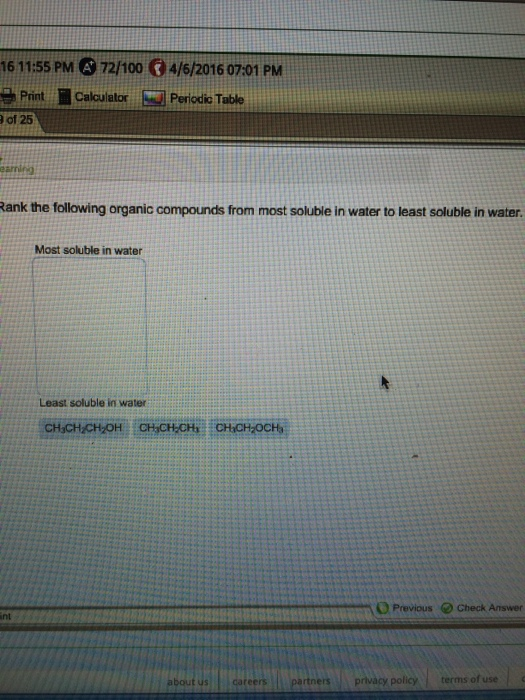Rank the following organic compounds from most soluble in water to least scluble in water.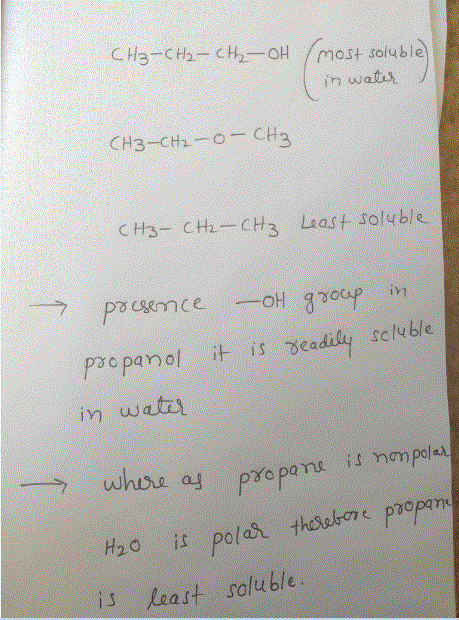##### Add Answer of: Rank the following organic compounds from most soluble in water to least scluble in water.
Similar Homework Help Questions
• ### Rank the following organic compounds from most soluble in water to least soluble in water. Question 5 of 11 Map Sapling...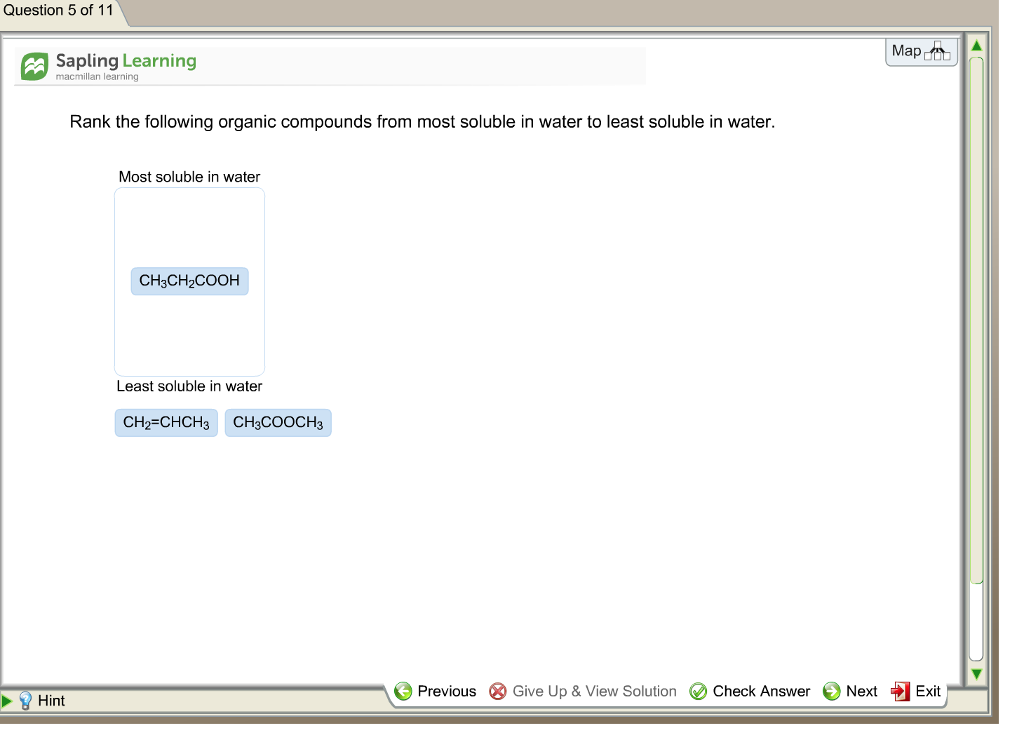Rank the following organic compounds from most soluble in water to least soluble in water. Question 5 of 11 Map Sapling Learning macmillan learning Rank the following organic compounds from most soluble in water to least soluble in water. Most soluble in water CH3CH2COOH Least soluble in water CH2=CHCH3 CH3COOCH3 毛Previous 8) Give Up & View Solution (V) Check Answer 0 Next +1 Exit Hint

• ### Rank the following compounds from most soluble in water to least soluble in water. Rank the following compounds from mo...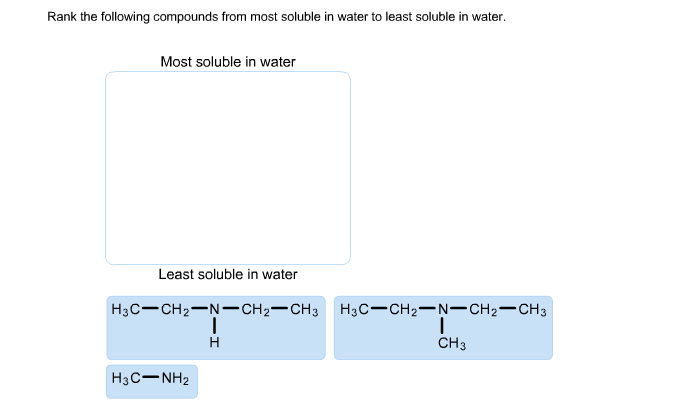Rank the following compounds from most soluble in water to least soluble in water. Rank the following compounds from most soluble in water to least soluble in water Most soluble in water Least soluble in water H3C- CH2-N- CH2CH3 H3C CH2 N-CH2 CH3 CH3 H3C-NH2

• ### Rank the following organic compounds from most soluble in water to least soluble in water. CH3CH2OCH3 CH3CH2CH3 CH3CH2CH...

Rank the following organic compounds from most soluble in water to least soluble in water. CH3CH2OCH3 CH3CH2CH3 CH3CH2CH2OH

• ### Rank the following organic compounds from most soluble in water to least soluble in water. a.CH3OCH3 b.CH3CH3 c. CH3...

Rank the following organic compounds from most soluble in water to least soluble in water. a.CH3OCH3 b.CH3CH3 c. CH3CH2OH

• ### es and Due Dates Ch 11 Guided Inquiry OR Arrange these solutes from most soluble in water to least soluble in water...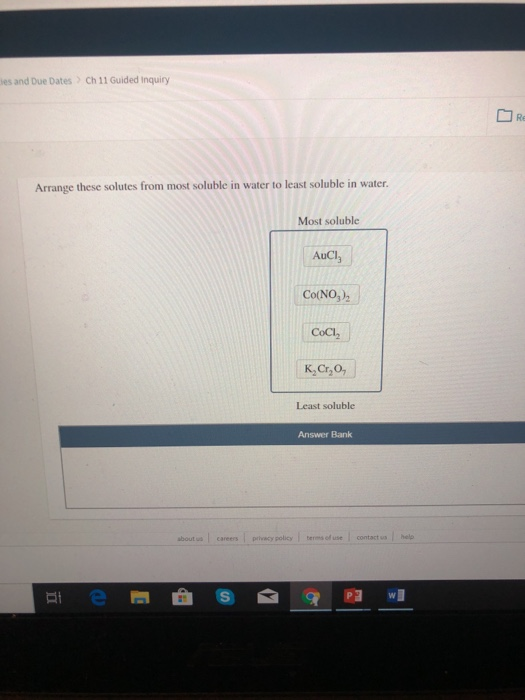es and Due Dates Ch 11 Guided Inquiry OR Arrange these solutes from most soluble in water to least soluble in water. Most soluble AUCI Co(NO3)2 СоСІ, K₂Cr₂O, Least soluble Answer Bank

• ### Rank the following from most to least acidic. Rank from most to least acidic. To rank items as equivalent, overlap...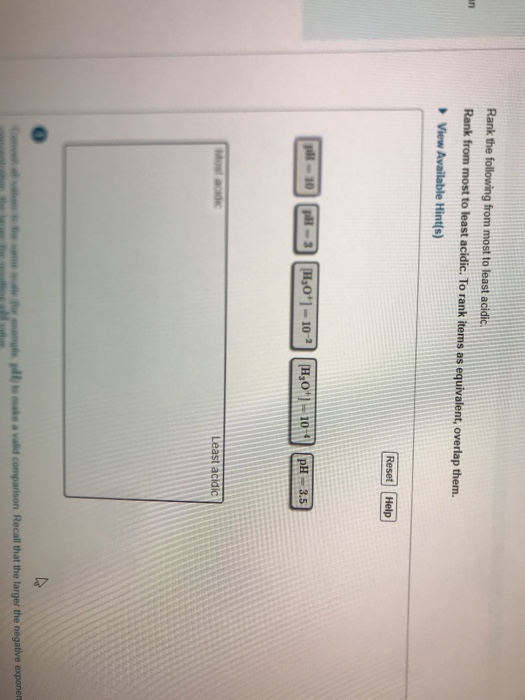Rank the following from most to least acidic. Rank from most to least acidic. To rank items as equivalent, overlap them. View Available Hint(s) Reset Help 1-2 DH (1:0*) – 102 1,0') 2009 - 33 Least acidic w w w .comparison Recall that the larger the negative exponen

• ### 1. (2 pts) Rank the compounds in order of increasing acidity (1 = least acidic, 4 = most acidic)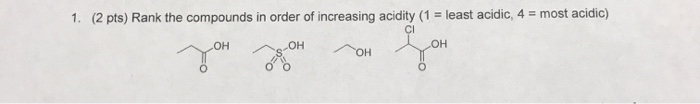1. (2 pts) Rank the compounds in order of increasing acidity (1 = least acidic, 4 = most acidic)

• ### 4. Rank the following compounds from least to most basic. V. iv. lii il. NH2 A....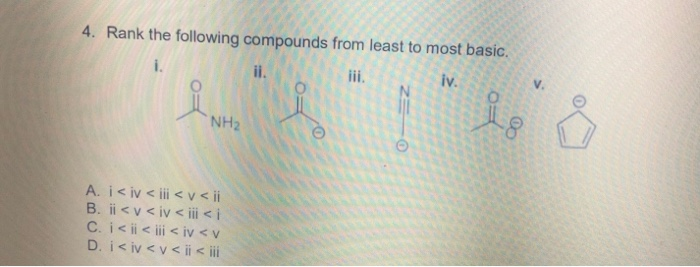4. Rank the following compounds from least to most basic. V. iv. lii il. NH2 A. i< iv < iii< v< i B. ii< v< iv < ii < i C. i< ii< ii < iv <v D. i< iv<v<ii < ii 4. Rank the following compounds from least to most basic. V. iv. lii il. NH2 A. i

• ### Rank the following carbocations in order of decreasing stability.

Rank the following carbocations in order of decreasing stability.

• ### Rank the following carbocations in order of decreasing stability.

Rank the following carbocations in order of decreasing stability.

Need Online Homework Help?